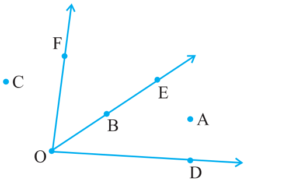# NCERT Class 6 Mathematics Fourth Chapter Basic Geometrical Ideas Exercise 4.3 Solutions

## EXERCISE 4.3

(1) Name the angles in the given figure.Ans: ∠DAB; ∠ABC; ∠BCD; ∠CDA

(2) In the given diagram, name the point(s)(a) In the interior of ∠DOE

Ans: A

(b) In the exterior of ∠EOF

Ans: A, C, D

(c) On ∠EOF

Ans: E, B, O, F

(3) Draw rough diagrams of two angles such that they have,

(a) One point in common.∠COD and ∠AOB have point O in common.

(b) Two points in common.∠AOB and ∠BOC have points O and B in common.

In case you are missed :- Previous Chapter Exercise Solution

(c) Three points in common.∠AOB and ∠BOC have points O, E, B in common.

(d) Four points in common.∠BOA and ∠COA have points O, E, D, A in common.

(e) One ray in common.In case you are missed :- Next Chapter Exercise Solution

Updated: April 14, 2023 — 1:45 pm